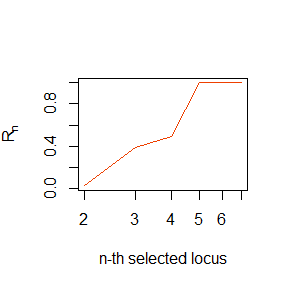# Tutorial for the R MMD package

The MMD package was developed in . This tutorial illustrates the functioning of the MMD package using Campylobacter isolates from human and five animal sources (Cattle, Chicken, Pig, Sheep, and WB). For illustration purposes, the example uses genotypes of reduced size consisting 10 isolates per host reservoir described with genotypes of 10 SNPs. The data used in this tutorial comes with the MMD package. Other datasets studied in Ref.  are available from this link . To use these examples, simply download the data from the link.

## Source attribution

### Setting the parameters for source attribution

Set the file names of the file csv containing genotype data and file pop containing population names used in this example:

datafile <- system.file("extdata", "Campylobacter_10SNP_HlW.csv", package = "MMD")
popfile <- system.file("extdata", "Campylobacter_10SNP_HlW.pop", package = "MMD")

Note that the full path to the files should be specified. For example:

datafile
##  "C:/Users/s05fp2/AppData/Local/Temp/Rtmp0w5QXw/Rinstf2c33403434/MMD/extdata/Campylobacter_10SNP_HlW.csv"
popfile
##  "C:/Users/s05fp2/AppData/Local/Temp/Rtmp0w5QXw/Rinstf2c33403434/MMD/extdata/Campylobacter_10SNP_HlW.pop"

This example searches for the data from the MMD package which is found using system.file. In general, however, in this step you should specify the path to the directory where you have stored the data you want to analyse.

Set the name of the population to be attributed. In this case, we want to attribute Campylobacter isolates from “Human”:

ToAttribute <- "Human"

Introduce the population names of the genotypes to be used as sources (these are available from the file *.pop):

sourcenames <- c("Cattle","Chicken","Pig","Sheep","WB")

Set the maximum number of loci to be used in the analysis:

NL <- 100

NL was set to 100 loci as an example but the program will use 10 loci since the genotypes in the file Campylobacter_10SNP_HlW.csv consist of 10 SNPs.

### Running the function MMD_attr for source attribution (verbose=FALSE by default even if verbose is not specified; set verbose=TRUE to see a progress bar for computations if you wish):

attribution <- MMD_attr(datafile,popfile,NL,sourcenames,ToAttribute,verbose=FALSE)

### Source attribution results:

In this example, the source attribution results were stored in attribution:

attribution

The list contains 7 items:

• Number of individuals of unknown origin (Campylobacter isolates from humans):
[]
 500

• Number of sources:
[]
 5

• Statistics of the attribution probability to sources, ps:
[]
Sources mean standard.deviation X2.5.percentile X97.5.percentile
1 Cattle 0.28475914 0.003788603 0.27715866 0.29197614
2 Chicken 0.32152237 0.004583734 0.31249737 0.33048377
3 Pig 0.05004946 0.007727446 0.03526279 0.06562583
4 Sheep 0.24744750 0.001905219 0.24357132 0.25093940
5 WB 0.09623947 0.006093308 0.08474618 0.10863976

• Runtime of the calculation:
[]
Time difference of 1.759793 mins

• Probability pu,s that each individual of unknown origin is attributed to sources:
[]
individual Cattle Chicken Pig Sheep WB
1 301 0.317562288 0.36062158 0.0000000 0.2610470 0.06076916
2 302 0.317562288 0.36062158 0.0000000 0.2610470 0.06076916
3 303 0.317562288 0.36062158 0.0000000 0.2610470 0.06076916
4 304 0.317562288 0.36062158 0.0000000 0.2610470 0.06076916
5 305 0.192435285 0.01012817 0.3155315 0.2532043 0.22870068
6 306 0.317562288 0.36062158 0.0000000 0.2610470 0.06076916
7 307 0.317562288 0.36062158 0.0000000 0.2610470 0.06076916
…..

• Number of loci:
[]
 10

• Parameter q used to calculate the q-quantile of the Hamming distance in the MMD method. Since a value was not specified for the MMD_attr function, the default value was used:
[]
 0.01

For example, a bar chart for the mean of the probability of attribution of Campylobacter human isolates to food sources can be easily obtained from attribution as follows:

barplot(height = attribution[]$mean,names.arg = attribution[]$Sources,las=2,cex.names = 0.8,main=NULL, ylab = expression("Attribution probability, p"[s]))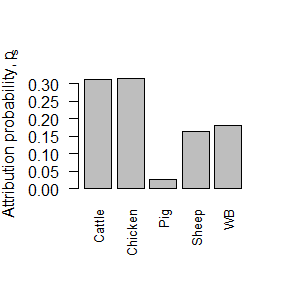## Self-attribution

This example illustrates self-attribution of Campylobacter isolates from the pig reservoir of the dataset used above. The genotypes from pig are split into training and test datasets. The test dataset is defined by randomly drawing 50% of the isolates from pig reservoir; the remaining isolates define the training dataset.

### Setting the parameters

To run self-attribution, the function MMD_attr can be executed as follows:

SelfAttribution <- MMD_attr(datafile,popfile,NL,sourcenames,ToAttribute = "Pig",SelfA = "yes",optq = "yes", pqmin = 0, pqmax = 0.5, np=1000, fSelfA = 0.5)

Here,
* ToAttribute is set to the “Pig” reservoir which will be analysed for self-attribution.
* SelfA is set to “yes” to do self-attribution (SelfA is set to “no” by default in the function MMD_attr, i.e. it is set by default to do source attribution instead of self-attribution).
* fSelfA is the fraction of genotypes in the “Pig” reservoir used to define the test dataset. Here, this is set to 0.5 which, in fact, is the default value.
* optq is set to “yes” to optimise the q-quantile for self-attribution accuracy (optq is set to “no” by default in the function MMD_attr).
* Optimisation of the q-quantile is done by considering a partition of np values in the interval [pqmin,pqmax] of the q-quantile. * The value of verbose was not explicitly indicated and the function will use the default value, verbose=FALSE, so that a progress bar will not be displayed.

### Self-attribution results

The results are stored in the list SelfAttribution:

SelfAttribution

The list contains 8 items:

• Number of individuals of unknown origin (pig Campylobacter isolates from the test dataset):
[]
 65

• Number of sources:
[]
 5

• Statistics of the attribution probability to sources, ps:
[]
Sources mean standard.deviation X2.5.percentile X97.5.percentile
1 Cattle 0.03104321 0.0042615251 0.02321769 0.03993305
2 Chicken 0.04086488 0.0008201976 0.03933067 0.04237244
3 Pig 0.70524955 0.0105881858 0.68546798 0.72476244
4 Sheep 0.16327327 0.0023348811 0.15896031 0.16814020
5 WB 0.05963771 0.0049012502 0.05068933 0.06987849

• Probability pu,s that each individual of unknown origin is attributed to sources:
[]
individual Cattle Chicken Pig Sheep WB
1 805 0.006715212 0.04700648 0.7593355 0.1544499 0.03249296
2 806 0.006715212 0.04700648 0.7593355 0.1544499 0.03249296
3 807 0.006715212 0.04700648 0.7593355 0.1544499 0.03249296
4 808 0.006715212 0.04700648 0.7593355 0.1544499 0.03249296
5 810 0.006715212 0.04700648 0.7593355 0.1544499 0.03249296
6 812 0.006715212 0.04700648 0.7593355 0.1544499 0.03249296
7 813 0.078421452 0.03136858 0.5881609 0.1882115 0.11383759
…..

• Runtime of the calculation:
[]
Time difference of 1.941194 mins

• Number of loci:
[]
 10

• Value of the q-quantile of the Hamming distance for which the self-attribution accuracy is maximised. Accuracy is the fraction of genotypes in the test dataset that are correctly attributed to the chosen reservoir (pig in this example):
[]
 0.219

• Self-attribution accuracy as a function of the q-quantile parameter:
[]
q.quantile prob..correct.attribution
1 0.0000 0.6337530
2 0.0005 0.6337530
…..

In this case, the bar chart for the probability that isolates of unknown origin are attributed to each of the sources can be obtained as follows:

barplot(height = SelfAttribution[]$mean,names.arg = SelfAttribution[]$Sources,las=2,cex.names = 0.8,main=NULL, ylab = expression("Attribution probability, p"[s]))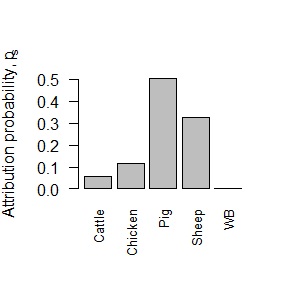Despite the small number of SNPs used in the example dataset, it is interesting that the program predicts a relatively high probability of attribution to the correct source of pig Campylobacter isolates.

## Selection of informative loci

The package allows informative loci to be selected in terms of entropy and redundancy measures.

### Entropy measures

MMD_Entropy provides entropy measures for individual loci. It can be called as follows:

EntropyLoci <- MMD_Entropy(datafile,popfile,NL,sourcenames)

Again, here you can specify verbose=TRUE to see a progress bar for computations if you wish.

#### Outputs of MMD_Entropy

The results give a list of 7 items:

EntropyLoci
• Number of loci used for the calculation:
[]
 10

• Number of individuals in sources:
[]
 673

• Proportional weight of each population, qs:
[]
Sources qs
[1,] “Cattle” “0.222882615156018”
[2,] “Chicken” “0.222882615156018”
[3,] “Pig” “0.193164933135215”
[4,] “Sheep” “0.222882615156018”
[5,] “WB” “0.138187221396731”

• Number of alleles in the dataset:
[]
 4

• Value of the alleles in the dataset:
[]
 1 2 3 4

• Data frame with three columns for entropies: Total (HlT), within-sources (HlW) and between-sources (HlB):
[]
HlT HlW HlB
1 0.01610117 0.01184854 0.004252624
2 1.24988869 0.63488510 0.615003590
3 0.91779974 0.49274008 0.425059661
4 0.91779974 0.49274008 0.425059661
….

• Runtime:
[]
Time difference of 0.06805801 secs

Using the list EntropyLoci one can easily plot histograms for the different entropies,

histHlT <- hist(EntropyLoci[]$HlT,col="#1C86EE",main=NULL,xlab=expression(paste("H"^"T")),ylab="Frequency")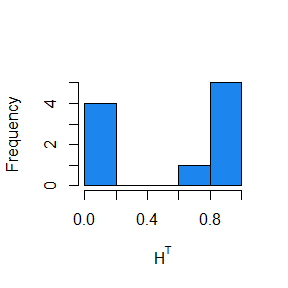histHlB <- hist(EntropyLoci[]$HlB,col="#1C86EE",main=NULL,xlab=expression(paste("H"^"B")),ylab="Frequency")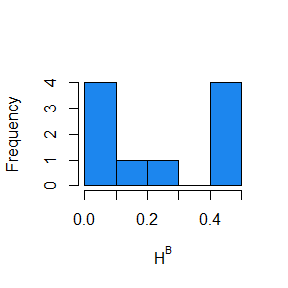histHlW <- hist(EntropyLoci[]$HlW,col="#1C86EE",main=NULL,xlab=expression(paste("H"^"W")),ylab="Frequency")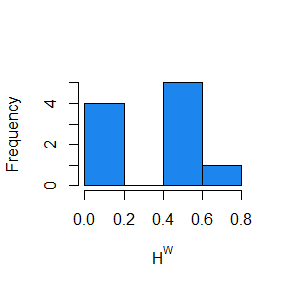or a scatterplot of the entropy between sources vs. entropy within sources for each locus: plot(cbind(EntropyLoci[]$HlW,EntropyLoci[]\$HlB),col="#1C86EE",main=NULL, xlab=expression(paste("H"^"W")),ylab=expression(paste("H"^"B")))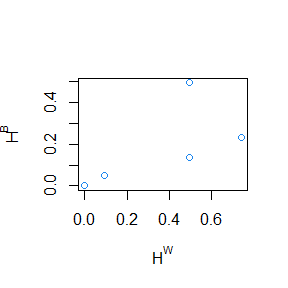### Redundancy of loci

In a sequential selection of loci, the redundancy of the n-th locus can be calculated using the funtion MMD_Rn as follows:

RedundancyLoci <- MMD_Rn(datafile,popfile,NL,sourcenames)

#### Outputs of MMD_Rn

The results give a list of 9 items:

RedundancyLoci
• Number of loci used for the calculation:
[]
 10

• Number of individuals in sources:
[]
 673

• Proportional weight of each population, qs:
[]
Sources qs
[1,] “Cattle” “0.222882615156018”
[2,] “Chicken” “0.222882615156018”
[3,] “Pig” “0.193164933135215”
[4,] “Sheep” “0.222882615156018”
[5,] “WB” “0.138187221396731”

• Number of alleles in the dataset:
[]
 4

• Value of the alleles in the dataset:
[]
 1 2 3 4

• Data frame with redundancy Rn of each locus:
[]
locus Rn_original
1 2 0.001799635
2 3 0.889203483
3 4 1.000000000
….

• Index of loci with increasing Rn:
[]
 1 5 9 8 6 3 2 4 7

• Data frame with redundancy Rn of loci after sorting in increasing order:
[]
locus Rn_sorted
1 2 0.001793803
2 3 0.002553717
3 4 0.160958703
4 5 0.271356486
….

• Runtime:
[]
Time difference of 0.13464 secs

For example, one can plot the redundancy Rn of loci as a function of n and the redundacy of loci sorted in increasing order:

plot(RedundancyLoci[],col="#EE4000",main=NULL,log="x",type="l",xlab="n-th selected locus",ylab=expression("R"[n]))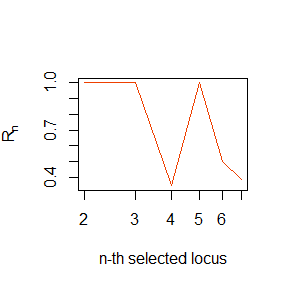plot(RedundancyLoci[],col="#EE4000",main=NULL,log="x",type="l",xlab="n-th selected locus",ylab=expression("R"[n]))